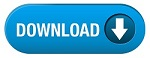Home » SSC » Model Questions for Scientific Assistant (IMD) Exam (Paper-2) PHYSICS – Set 6

a. Free fall
b. Mass
c. Weight
d. Kilogram

a. Inertia
b. Force
c. Weight
d. Kilogram

a. Inertia
b. Mass
c. Weight
d. Kilogram

a. Inertia
b. Mass
c. Weight
d. Newton

a. Inertia
b. Mass
c. Weight
d. Volume

#a. Inertia
b. Mass
c. Weight
d. Kilogram

a. Inertia
b. Mass
c. Weight
d. Kilogram

a. Inertia
b. Mass
c. Weight
d. Kilogram

## 9. The force of gravity acting on a 100 kg person is ___ compared to the force of gravity on a 1 kg book

a. 4 times larger
b. the same
c. 100 times larger
d. lower

## 10. There is only one force acting on an object. For the object to travel at constant velocity, this force must be—-

a. a constant non-zero value
b. a varying non-zero value
c. a constant zero value
d. a positive value

#